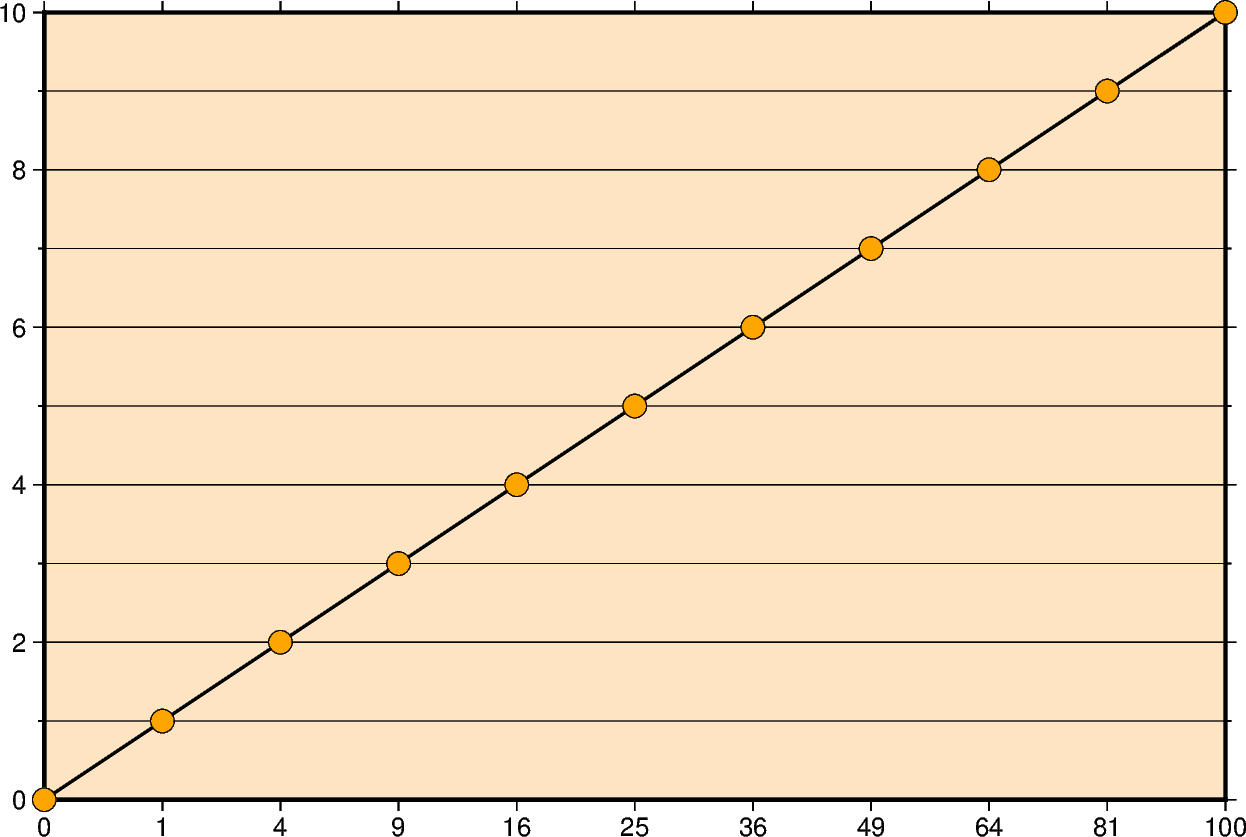# Cartesian power

Xwidth[ppvalue][/height[ppvalue]] or xx-scale[ppvalue][/y-scale[ppvalue]]

Give the width of the figure and the optional argument height. The lower-case version x is similar to X but expects an x-scale and an optional y-scale. Each axis with a power transformation requires p and the exponent for that axis after its size argument.```import numpy as np
import pygmt

# Create a list of y-values 0-10
yvalues = np.arange(0, 11)
# Create a list of x-values that are the square of the y-values
xvalues = yvalues**2

fig = pygmt.Figure()
fig.plot(
region=[0, 100, 0, 10],
# Set the power transformation of the x-axis, with a power of 0.5
projection="X15cp0.5/10c",
# Set the figures frame and color as well as
# annotations and ticks
# The "p" forces to show only square numbers as annotations
# of the x-axis
frame=["WSne+givory", "xa1p", "ya2f1"],
# Set the line thickness to "thick" (equals "1p", i.e. 1 point)
# Use as color "black" (default) and as style "solid" (default)
pen="thick,black,solid",
x=xvalues,
y=yvalues,
)
# Plot x-, y-values as points on the line
# Style of points is 0.2 cm circles, color fill is "green" with a "black"
# outline. Points are not clipped if they go off the figure
fig.plot(x=xvalues, y=yvalues, style="c0.2c", fill="green", no_clip=True, pen="black")
fig.show()
```

Total running time of the script: (0 minutes 0.684 seconds)

Gallery generated by Sphinx-Gallery